# Developing methods for identifying the inflection point of a convex/concave curve

## The Fisher-Pry sigmoid curve with total symmetry (not noisy)

Let’ s take the function: $f (x) = 5 + 5\, tanh (x − 5)$ after , which has p = 5, L = 10, x1 = 2.7024, x99 = 7.2976 and examine it at the interval [2, 8] in order to have data symmetry w.r.t. inflection point. The function is also symmetrical around inflection point, i.e. we have total symmetry.

From Corollary 1.1 of  we compute $$x_l = 5.970315941, x_r = 4.029684059$$, $$x_{F1} = 3.850750196, x_{F2} = 6.149249804$$, all inside [2, 8], thus all methods are theoretically applicable.

We first take n = 500 sub-intervals equal spaced without error just for checking our estimators. The results are presented at Table 1 of , while here we also present the BESE iterations done by ‘bese()’.

library(inflection)
data("table_01")
x=table_01$x y=table_01$y
plot(x,y,cex=0.3,pch=19)
grid()
bb=ese(x,y,0);bb
##      j1  j2 chi
## ESE 170 332   5
pese=bb[,3];pese
##  5
abline(v=pese)
cc=bese(x,y,0)
cc$iplast ##  5 abline(v=cc$iplast,col='blue')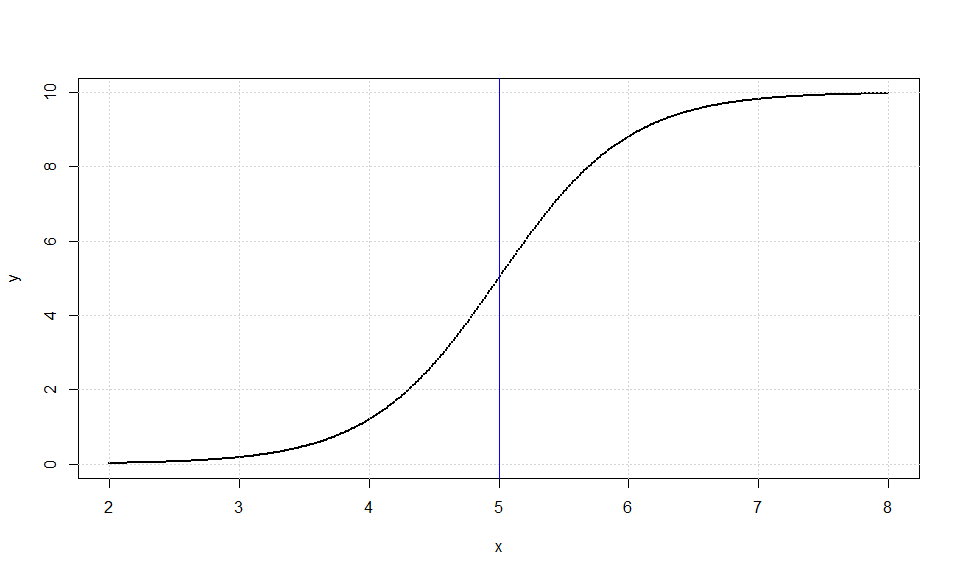knitr::kable(cc$iters, caption = 'BESE') BESE n a b ESE 501 2.000 8.000 5 163 4.556 5.444 5 75 4.784 5.216 5 37 4.892 5.108 5 19 4.952 5.048 5 9 4.976 5.024 5 5 4.988 5.012 5 We observe that $$\chi_l = 5.9720, \chi_r = 4.0280$$, $$\chi_{F1} = 3.8480, \chi_{F2} =6.1520$$ are very close to the theoretically expected values, so we are on the results of Lemma 1.3 of . The absolutely accuracy from the first apply of all methods confirms our theoretical analysis. ## The Fisher-Pry sigmoid curve with total symmetry (noisy) We next add the error term $$\epsilon_i\sim\,U(−0.05, 0.05)$$ via the process 14 of  and run our algorithms again.The results are presented at Table 2 of  and here we also present the BESE iterations done by ‘bese()’. library(inflection) data("table_02") x=table_01$x
y=table_01$y plot(x,y,cex=0.3,pch=19) grid() bb=ese(x,y,0);bb ## j1 j2 chi ## ESE 170 332 5 pese=bb[,3];pese ##  5 abline(v=pese) cc=bese(x,y,0) cc$iplast
##  5
abline(v=cc$iplast,col='blue')knitr::kable(cc$iters, caption = 'BESE')
BESE
n a b ESE
501 2.000 8.000 5
163 4.556 5.444 5
75 4.784 5.216 5
37 4.892 5.108 5
19 4.952 5.048 5
9 4.976 5.024 5
5 4.988 5.012 5

## The Fisher-Pry sigmoid curve with data left asymmetry (not noisy)

We continue with the same sigmoid function, but now we choose a proper [a, b] to show data asymmetry w.r.t. inflection point.

Let’ s take for example [4.2, 8]. If we do our theoretical computations we find $$x_l = 5.974322740, x_r = 4.029684059$$, $$x_{F1} = 4.025677260$$, $$x_{F2} = 5.974322740$$. We have that $$x_r < a$$, so $$\chi_r$$ has to estimate a = 4.2 and $$\chi_S$$ must be close to 4.703504993. Additionally, $$x_{F1} < a$$, so $$\chi_{F1}$$ must be also an estimation of a, thus $$\chi_{D}$$ must lie near the value 5.087161370. It’ s time to see if our theoretical predictions will be confirmed by experiment. We use for comparability the same Standard Partition as before and have the output presented at Table 3 and 4 of .

data("table_03_04")
x=table_03_04$x y=table_03_04$y
tese=ese(x,y,0);tese
##     j1  j2    chi
## ESE  2 156 4.7928
pese=tese[,3]
tede=ede(x,y,0);tede
##     j1  j2    chi
## EDE  1 234 5.0854
pede=tede[,3]
cc=bese(x,y,0)
cc$iplast ##  5.0018 dd=bede(x,y,0) dd$iplast
##  4.998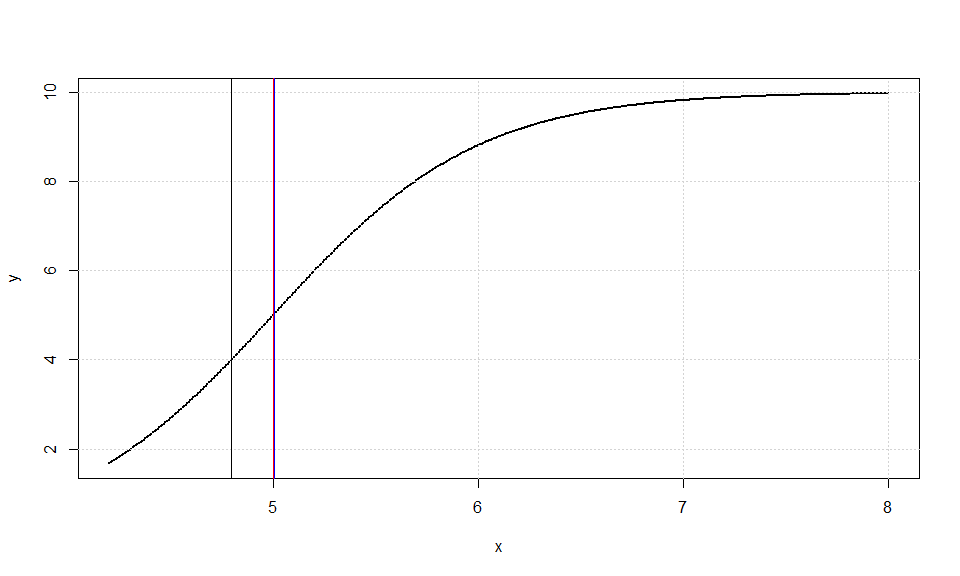BESE
n a b ESE
501 4.2000 8.0000 4.7928
155 4.8156 5.3704 5.0930
74 4.8232 5.0892 4.9562
36 4.9524 5.0816 5.0170
18 4.9600 5.0208 4.9904
9 4.9904 5.0132 5.0018
BEDE
n a b EDE
501 4.2000 8.0000 5.0854
234 4.5192 5.4844 5.0018
128 4.7244 5.2716 4.9980
73 4.8460 5.1576 5.0018
42 4.9068 5.0892 4.9980
25 4.9448 5.0512 4.9980
15 4.9676 5.0284 4.9980
9 4.9828 5.0208 5.0018
6 4.9904 5.0132 5.0018
4 4.9904 5.0056 4.9980

## The Fisher-Pry sigmoid curve with data left asymmetry (noisy)

Let’ s add again an same error term $$\epsilon_i\sim\,U(−0.05, 0.05)$$ and run our algorithms. The results at Table 5 of  clearly are close enough to the theoretical expectations. Since ESE method did not estimate the inflection point with acceptable accuracy, after running BESE and BEDE iterative methods we find Table 6 of  which is a clear improvement of both estimations.

data("table_05_06")
x=table_05_06$x y=table_05_06$y
tese=ese(x,y,0);tese
##     j1  j2  chi
## ESE  3 149 4.77
pese=tese[,3]
tede=ede(x,y,0);tede
##     j1  j2    chi
## EDE  3 231 5.0816
pede=tede[,3]
cc=bese(x,y,0)
cc$iplast ##  5.036 dd=bede(x,y,0) dd$iplast
##  4.9828
plot(x,y,cex=0.3,pch=19)
grid()
abline(v=pese)
abline(v=cc$iplast,col='blue') abline(v=dd$iplast,col='red')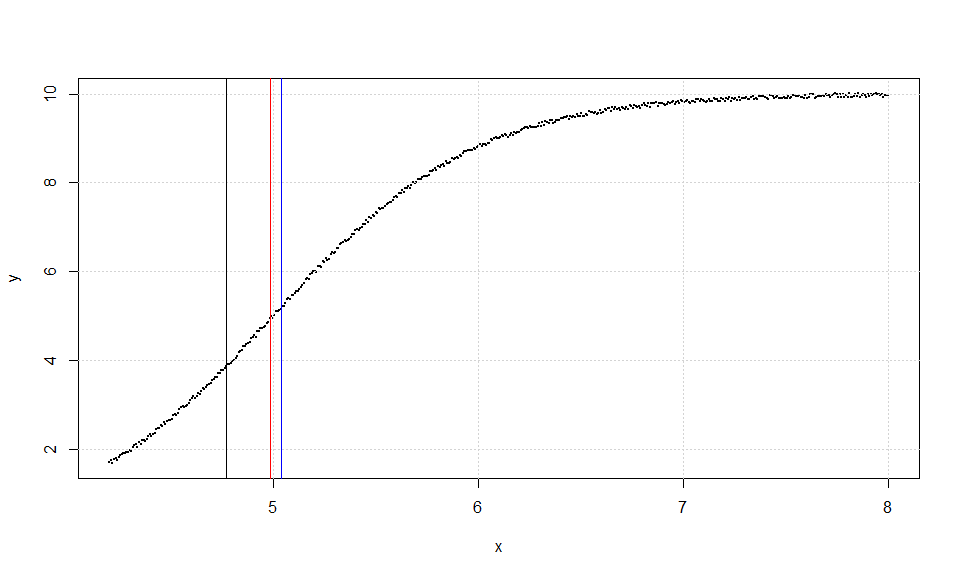knitr::kable(cc$iters, caption = 'BESE') BESE n a b ESE 501 4.2000 8.0000 4.7700 147 4.8156 5.2792 5.0474 62 4.9144 5.1576 5.0360 knitr::kable(dd$iters, caption = 'BEDE')
BEDE
n a b EDE
501 4.2000 8.0000 5.0816
229 4.5268 5.5148 5.0208
131 4.7244 5.2412 4.9828

## The Gompertz non symmetric sigmoid curve (not noisy)

Let’ s examine the function: $f (x) = 10 e^{-e^{5}e^{−x}}$ after , in the interval [3.5, 8]. It is easy to prove that f is (0.224, 1.0)-asymptotically symmetric around inflection point, so we can handle it similar to a symmetric sigmoid only for a distance of ±1 from p = 5.

We use, for comparison reasons, the same SP with 500 sub-intervals without error and obtain the Table 8 of  which is absolutely compatible with theoretical predictions. The ESE & EDE iterations are showed at Table 9 of  where we observe convergence to the real p for both two methods.

data("table_08_09")
x=table_08_09$x y=table_08_09$y
tese=ese(x,y,0);tese
##     j1  j2   chi
## ESE 72 266 5.012
pese=tese[,3]
tede=ede(x,y,0);tede
##     j1  j2   chi
## EDE 67 311 5.192
pede=tede[,3]
cc=bese(x,y,0)
cc$iplast ##  4.9985 dd=bede(x,y,0) dd$iplast
##  4.9985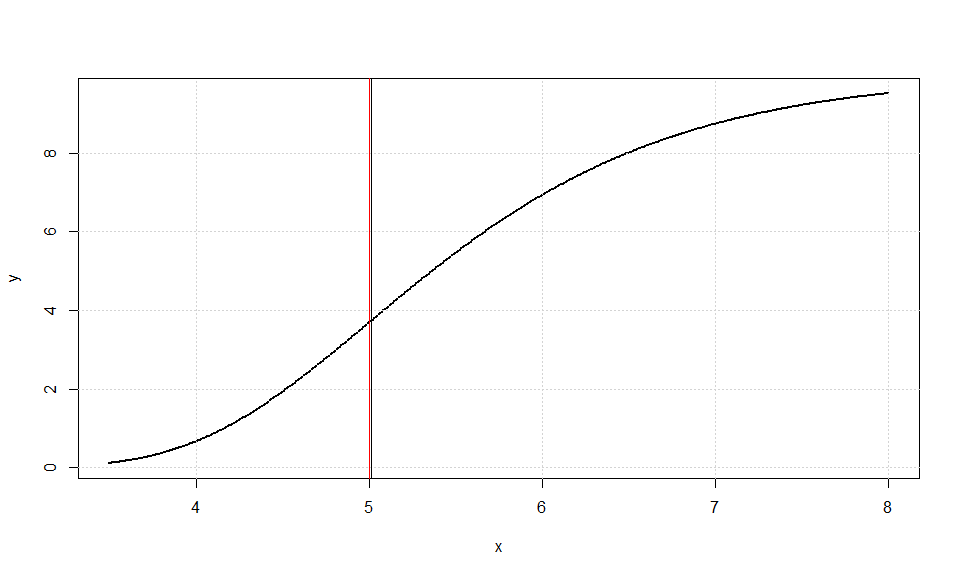BESE
n a b ESE
501 3.500 8.000 5.0120
195 4.625 5.489 5.0570
97 4.778 5.201 4.9895
48 4.904 5.120 5.0120
25 4.940 5.048 4.9940
13 4.976 5.030 5.0030
7 4.985 5.012 4.9985
BEDE
n a b EDE
501 3.500 8.000 5.1920
245 4.454 5.669 5.0615
136 4.670 5.363 5.0165
78 4.805 5.210 5.0075
46 4.886 5.120 5.0030
27 4.931 5.066 4.9985
16 4.958 5.039 4.9985
10 4.976 5.021 4.9985
6 4.985 5.012 4.9985

## The Gompertz non symmetric sigmoid curve (noisy)

We continue with our familiar SP by adding error uniformly distributed by U(−0.05, 0.05) and the results are given at Table 10 of  while ESE & EDE iterations are shown at Table 11 of .

data("table_10_11")
x=table_08_09$x y=table_08_09$y
tese=ese(x,y,0);tese
##     j1  j2   chi
## ESE 72 266 5.012
pese=tese[,3]
tede=ede(x,y,0);tede
##     j1  j2   chi
## EDE 67 311 5.192
pede=tede[,3]
cc=bese(x,y,0)
cc$iplast ##  4.9985 dd=bede(x,y,0) dd$iplast
##  4.9985BESE
n a b ESE
501 3.500 8.000 5.0120
195 4.625 5.489 5.0570
97 4.778 5.201 4.9895
48 4.904 5.120 5.0120
25 4.940 5.048 4.9940
13 4.976 5.030 5.0030
7 4.985 5.012 4.9985
BEDE
n a b EDE
501 3.500 8.000 5.1920
245 4.454 5.669 5.0615
136 4.670 5.363 5.0165
78 4.805 5.210 5.0075
46 4.886 5.120 5.0030
27 4.931 5.066 4.9985
16 4.958 5.039 4.9985
10 4.976 5.021 4.9985
6 4.985 5.012 4.9985

From these Tables we conclude that convergence to the true value of inflection point p = 5 occurs from the iterative application of ESE and EDE methods in one or two steps only.

## A symmetric 3rd order polynomial with total symmetry

Let the polynomial function: $f(x)=-\frac{1}{3}\,x^3+\frac{5}{2}\,x^2-4x+\frac{1}{2}$ We study it at [-2, 7], it has inflection point at p = 2.5 and we have total symmetry. The SP with 500 sub-intervals without error gives Table 13 of  which is absolutely compatible with theoretical predictions. There is no need for any kind of iteration, because both methods agree with the true value.

data("table_13")
x=table_13$x y=table_13$y
plot(x,y,cex=0.3,pch=19)
grid()
bb=ese(x,y,0);bb
##      j1  j2 chi
## ESE 126 376 2.5
pese=bb[,3];pese
##  2.5
abline(v=pese)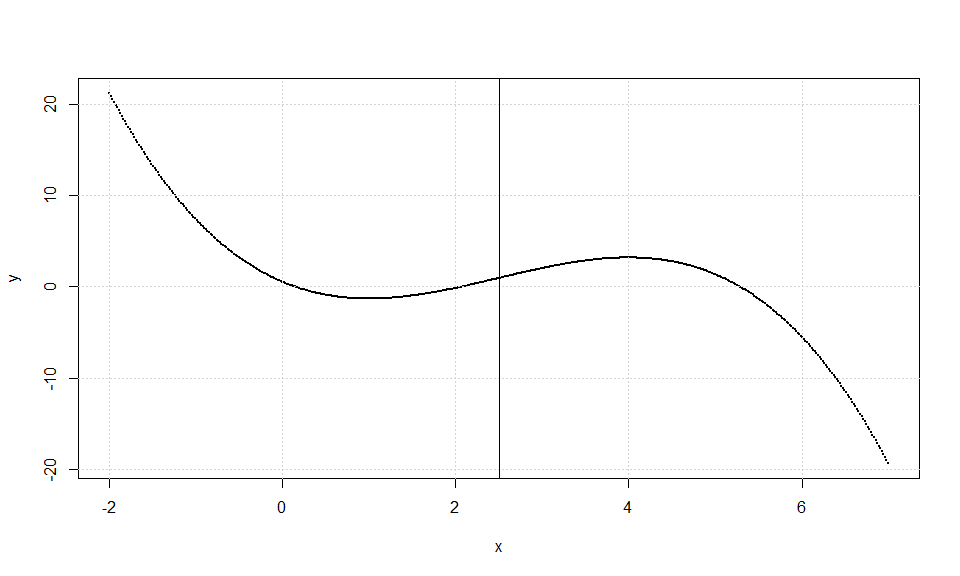The same SP with uniform error distributed by U(−2, 2) gives the results of Table 14 of  and two ESE iterations are presented at Table 15 of .

data("table_14_15")
x=table_14_15$x y=table_14_15$y
plot(x,y,cex=0.3,pch=19)
grid()
bb=ese(x,y,0);bb
##      j1  j2   chi
## ESE 115 375 2.392
pese=bb[,3];pese
##  2.392
abline(v=pese)
cc=bese(x,y,0)
cc$iplast ##  2.473 abline(v=cc$iplast,col='blue')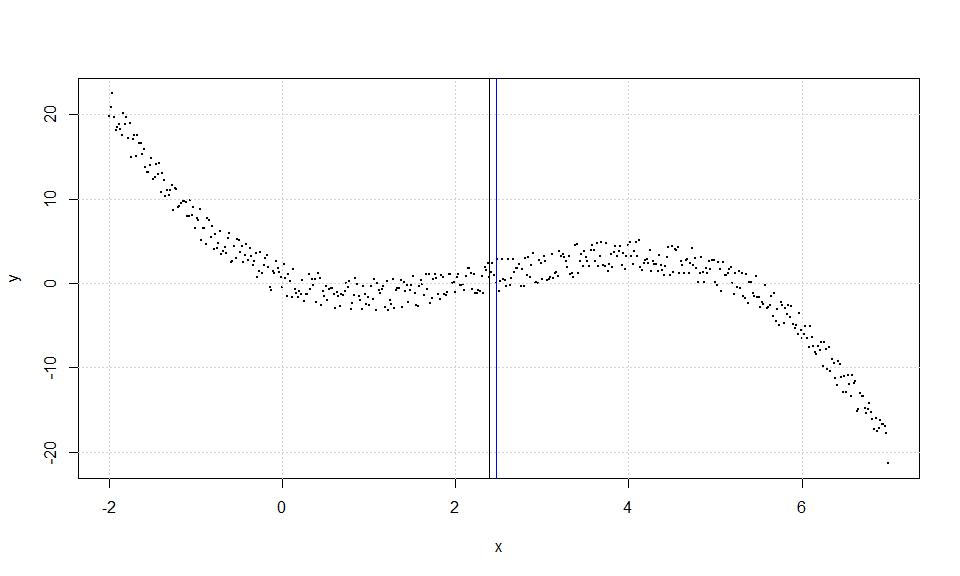knitr::kable(cc$iters, caption = 'BESE') BESE n a b ESE 501 -2.000 7.000 2.392 261 1.222 3.688 2.455 138 1.564 3.382 2.473 ## A symmetric 3rd order polynomial with data right asymmetry For the same symmetric 3rd order polynomial as above we change the interval to [-2, 8], thus we have data right asymmetry now. The case of SP with 500 sub-intervals and no error gives Table 17 of , while ESE and EDE iterations are presented at Table 18 of . First results are absolutely compatible with theoretical predictions for ESE method. data("table_17_18") x=table_17_18$x
y=table_17_18$y bb=ese(x,y,0);bb ## j1 j2 chi ## ESE 88 338 2.24 pese=bb[,3];pese ##  2.24 plot(x,y,cex=0.3,pch=19) grid() cc=bese(x,y,0) cc$iplast
##  2.5
dd=bede(x,y,0)
dd$iplast ##  2.5 abline(v=pese) abline(v=cc$iplast,col='blue')
abline(v=dd$iplast,col='red')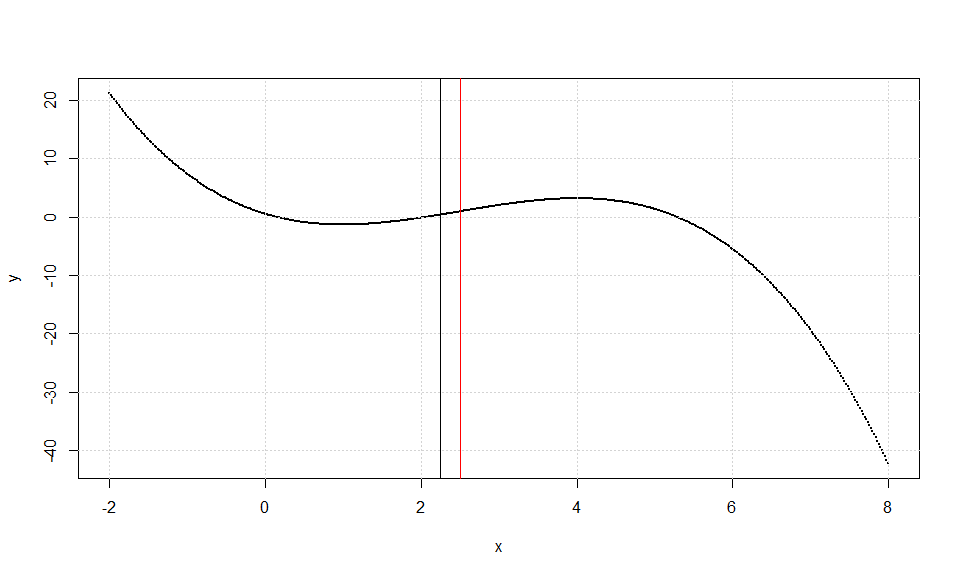knitr::kable(cc$iters, caption = 'BESE')
BESE
n a b ESE
501 -2.00 8.00 2.24
251 1.38 3.88 2.63
126 1.80 3.06 2.43
64 2.22 2.86 2.54
33 2.32 2.64 2.48
17 2.42 2.58 2.50
9 2.46 2.54 2.50
5 2.48 2.52 2.50
knitr::kable(dd$iters, caption = 'BEDE') BEDE n a b EDE 501 -2.00 8.00 2.5 293 0.82 4.18 2.5 169 1.54 3.46 2.5 97 1.94 3.06 2.5 57 2.18 2.82 2.5 33 2.32 2.68 2.5 19 2.40 2.60 2.5 11 2.44 2.56 2.5 7 2.46 2.54 2.5 5 2.48 2.52 2.5 We add uniform error distributed by U(-2, 2) and we have the results of Table 19 of , while one ESE & one EDE iteration are given at Table 20 of . data("table_19_20") x=table_19_20$x
y=table_19_20$y bb=ese(x,y,0);bb ## j1 j2 chi ## ESE 88 338 2.24 pese=bb[,3];pese ##  2.24 plot(x,y,cex=0.3,pch=19) grid() cc=bese(x,y,0) cc$iplast
##  2.65
dd=bede(x,y,0)
dd$iplast ##  2.35 abline(v=pese) abline(v=cc$iplast,col='blue')
abline(v=dd$iplast,col='red')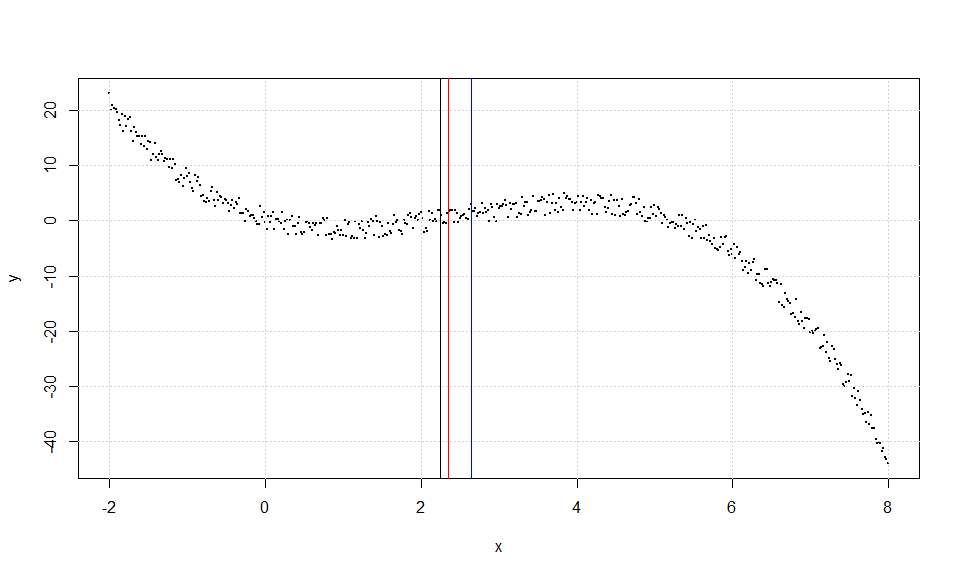knitr::kable(cc$iters, caption = 'BESE')
BESE
n a b ESE
501 -2.00 8.00 2.24
251 1.46 3.84 2.65
knitr::kable(dd\$iters, caption = 'BEDE')
BEDE
n a b EDE
501 -2.00 8.00 2.70
297 0.86 3.84 2.35

There exist a problem here. Although we have a symmetric polyno- mial, the TESE is not equal to the true inflection point. A remedy for this problem for the class of 3rd order polynomials is given with Lemma 2.1 of . Lets apply it here. We have that a = −2, b = 8 and from Table 19 of  is $$\chi_{r} = −0.26, \chi_{l} = 4.74$$, so we have that: $\hat{p}=\frac{1}{3}\,\chi_{l} + \frac{1}{3}\,\chi_{r}+\frac{1}{6}\,a+\frac{1}{6}\,b=2.493333333$ which is much closer to the true value of 2.5.